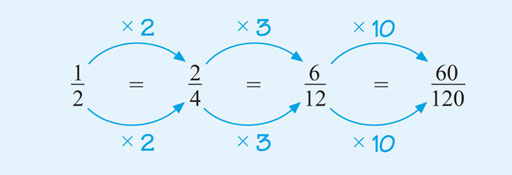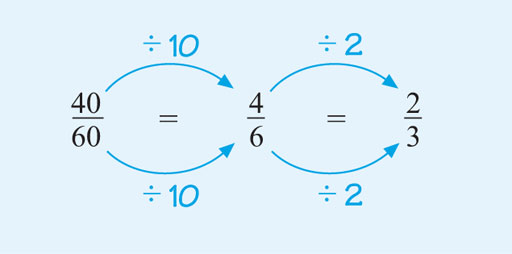Succeed with maths – Part 1

Start this free course now. Just create an account and sign in. Enrol and complete the course for a free statement of participation or digital badge if available.

Free course

# 2 Equivalent fractions

If you multiply the numerator (the number on top) and the denominator (the number underneath) of any fraction by the same number (except zero), you will get a fraction that is equivalent to the original one, as Figure 5 shows:Figure _unit4.2.1 Figure 5 Equivalent fractions by multiplying the numerator and denominator

Note that you must multiply the numerator and denominator by the same number. This is actually the same as multiplying by one, so it doesn’t change the value of the fraction. You can also generate equivalent fractions by dividing the numerator (top number) and the denominator (bottom number) of the fraction by the same number (again, not zero). This is the method that you will be employing frequently since fractions should usually be shown in their simplest form.

A fraction in its simplest form is one where you can no longer find a number (except one) to divide into both the numerator and the denominator to give you whole number answers.

This process of dividing the numerator (the number above the line) and the denominator (the number below the line) by the same number is known as cancelling or simplifying the fraction – hence ultimately a fraction in its simplest form.

Figure 6 shows an example of simplifying a fraction, using division. Before you look at the method used, think about how you would go about simplifying . Look for numbers that you can divide exactly into the top and bottom of the fraction.Figure _unit4.2.2 Figure 6 Equivalent fractions by dividing the numerator and the denominator

It is important to realise that there is often more than one way to simplify a fraction, so it is fine if you did use different steps here. For example, you could have started by dividing by 20, 5 or 2. The most efficient way in terms of the number of steps would be to start with 20 because you would need to use only one step.

Try these different starting steps for yourself now to see how else this could have been approached; just remember to divide the top and the bottom of the fraction by the same number. If there is no whole number apart from 1 that can be divided into both the numerator and the denominator, the fraction is said to be in its simplest form.

The next section shows a way of visualing this process.